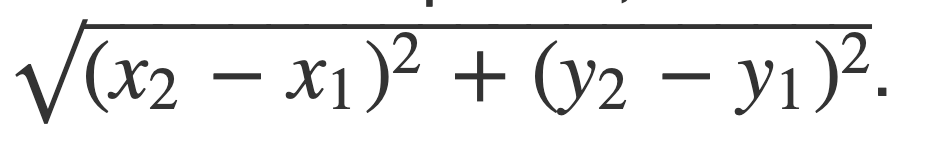# Ninja’s Service Center

Posted: 27 Mar, 2021
Difficulty: Moderate

## PROBLEM STATEMENT

#### Given a 2-D array ‘location’ where locations[ i ][ 0 ] and location[ i ][ 1 ] denotes the x and y coordinates of position of ‘i-th’ person.You need to return the minimum sum of Euclidian distance of Ninja’s service center from all customers.

The euclidian distance of a point [ x2, y2 ] from [ x1,y1 ] is given as##### Note :
``````You need to round up your answer to the nearest three decimal places.
``````
##### For Example :
``````If the position of the customers is [ [ 1,0 ], [ 4,0 ], [ 6,0 ] ], then if Ninja opens his service center at [ 4,0 ] then he will get the minimum sum of euclidian distance = 5.000  from all customers.
``````
##### Input Format :
``````The first line contains ‘T’ denoting the number of test cases.

The first line of each test case contains ‘N’ denoting the number of customers.

The next ‘N’ lines contain two space-separated integers ‘ x ’ and ‘ y ’ denoting the coordinates of the position of the customers.
``````
##### Output Format :
``````For each test case, print a single integer denoting the minimum sum of Euclidian distance.

Print the output of each test case in a separated line.
``````

#### Note :

``````You do not need to print anything, it has already been taken care of. Just implement the given function.
``````
##### Constraints :
``````1 <= T <= 10
1 <= N <= 10^3
0 <= location[ i ][ 0 ], location[ i ][ 1 ] <= 10^3

Where  location[ i ][ 0 ], location[ i ][ 1 ] denotes the ‘ x ’ and ‘ y ’ coordinates of the position of customers.

Time Limit : 1 sec
``````Approach 1

We will use the concept of the geometric median to solve this problem.

If we are given ‘N’ points in the 2-D plane then a point ‘p’ whose sum of distances from all the points in the 2-D plane is minimum is known as the geometric median.

This is exactly the same that we have to do to solve the problem. The approach to find the geometric median is as -

• Take a pair  ‘curPoint’ that will store the coordinates of a point and a variable ‘minDis’ that will store the minimum sum of distances from a point to all other points.
• We will first calculate the centroid of all the points and store this centroid point in ‘curPoint’ and store the sum of distances of this ‘centroid’ from all the points in ‘minDis’.
• Assume every customer’s position to be a geometric median and calculate the sum of the euclidian distance from all points. If any points give a minimum distance, update ‘curPoint’ and ‘minDis’.
• Now we will take a variable ‘shift’ and initialize it to some value let’s say 1000.
• We will move our ‘curPoint’ by this ‘shift’ value in all the four directions and if the  new points obtained in any of the four directions will give less value of the sum of distances than ‘minDis’ then we will update ‘curPoint’ and ‘minDis’
• If we do not get a point in any of the four directions that are minimizing our result, then we will divide the ‘shift’ value by 2 because we might have shifted too much and we need to look closer to the point.
• We will continue to add this ‘shift’ value until the shift value is greater than the value of minShift (let’s say minShift = 0.01).
• After this loop ends i.e ‘shift’ value becomes less than ‘minShift’ we will get the minimum sum of distances stored in ‘minDis’.

Algorithm

• Take a pair  ‘curPoint’ that will store the point that we are assuming the geometric median and a variable ‘minDis’.
• Find the coordinates of the centroid of all the points.
• curPoint = coordinates of centroid.
• minDis = sum of distances of centroid to all the points.
• Run a loop i : 0 to N - 1
• Calculate the sum of distances assuming location[ i ] be the geometric median.
• Update ‘minDis’ and ‘curPoint’ if a distance less than ‘minDis’ is obtained.
• Take a 2-D array ‘directions’ that will store move in all four directions.directions [ [ 0,1 ], [ 1, 0 ], [ -1, 0 ] , [ 0, -1 ] ].
• Take a variable ‘shift’ with an initial value = 1000 and a variable ‘minShift’ with an initial value of 0.01.
• Run a loop until shift > minShift
• Initialize a pair  ‘newPoint’ and a variable ‘flag’ with an initial value of 0.
• Run a loop i: 0 to 3 to check points in all four directions.
• newPoint.first = directions[ i ][ 0 ].
• newPoint.second = directions[ i ][ 1 ].
• Calculate the sum of the distance of ‘newPoint’ with all points.
• If this distance is less than ‘minDis’ then update ‘curPoint’ and ‘minDis’And make flag value 1.
• If flag = 0, it means we have not found any point that minimizes the sum of distance so divide ‘shift’ by 2.
• Return ‘minDis’.